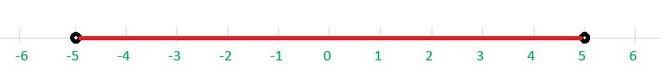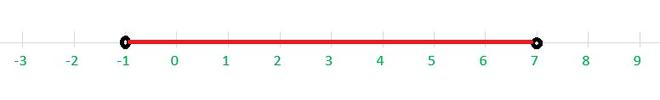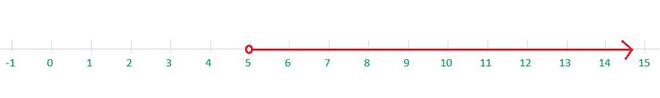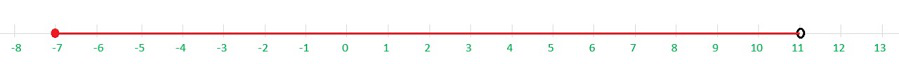# Class 11 NCERT Solutions- Chapter 6 Linear Inequalities – Miscellaneous Exercise on Chapter 6

• Difficulty Level : Hard
• Last Updated : 09 Mar, 2021

### Question 1. 2 ≤ 3x – 4 ≤ 5

Solution:

In this case, we have two inequalities, 2 ≤ 3x – 4 and 3x – 4 ≤ 5, which we will solve simultaneously.

We have 2 ≤ 3x – 4 ≤ 5

or 2 ≤ 3x – 4 and 3x – 4 ≤ 5

⇒ 2 + 4 ≤ 3x and 3x ≤ 5 + 4

⇒ 6 ≤ 3x and 3x ≤ 9

⇒≤ x and x ≤⇒ 2 ≤ x and x ≤ 3

⇒ 2 ≤ x ≤ 3

Hence, all real numbers x greater than or equal to 2 but less than or equal to 3 are solution of given 2 ≤ 3x – 4 ≤ 5 equality.

x ∈ [2, 3]

### Question 2. 6 ≤ – 3 (2x – 4) < 12

Solution:

In this case, we have two inequalities, 6 ≤ – 3 (2x – 4) and – 3 (2x – 4) < 12, which we will solve simultaneously.

We have 6 ≤ – 3 (2x – 4) < 12

or 6 ≤ – 3 (2x – 4) and – 3 (2x – 4) < 12

⇒≤ -(2x – 4) and -(2x – 4) <⇒ 2 ≤ -(2x – 4) and -(2x – 4) < 4

⇒ -2 ≥ (2x – 4) and (2x – 4) > -4   [multiplying the inequality with (-1) which changes the inequality sign]

⇒ -2+4 ≥ 2x and 2x > -4+4

⇒ 2 ≥ 2x and 2x > 0

⇒≥ x >⇒ 1≥ x > 0

⇒ 0 < x ≤ 1

Hence, all real numbers x greater than 0 but less than or equal to 1 are solution of given 6 ≤ – 3 (2x – 4) < 12 equality.

x ∈ (0, 1]

### Question 3. -3 ≤ 4-≤ 18

Solution:

In this case, we have two inequalities, -3 ≤ 4-and 4-≤ 18, which we will solve simultaneously.

We have -3 ≤ 4-≤ 18

or -3 ≤ 4-and 4-≤ 18

⇒ -3-4 ≤ –and –} ≤ 18-4

⇒ -7 ≤ –and –≤ 14

⇒ 7 ≥and≥ -14   [multiplying the inequality with (-1) which changes the inequality sign]

⇒ 7×2 ≥ 7x and 7x ≥ -14×2

⇒≥ x and x ≥⇒ 2 ≥ x ≥ -4

⇒ -4 ≤ x ≤ 2

Hence, all real numbers x greater than or equal to -4 but less than or equal to 2 are solution of given -3 ≤ 4-≤ 18 equality.

x ∈ [-4, 2]

### Question 4. -15 <≤ 0

Solution:

In this case, we have two inequalities, -15 <and} ≤ 0, which we will solve simultaneously.

We have -15 <≤ 0

or -15 <and≤ 0

⇒ -15×< (x-2) and (x-2) ≤ 0×⇒ -25 < x-2 and x-2 ≤ 0

⇒ -25+2 < x and x ≤ 0+2

⇒ -23 < x ≤ 2

Hence, all real numbers x greater than -23 but less than or equal to 2 are solution of given -15 ≤≤ 0 equality.

x ∈ (-23, 2]

### Question 5. -12 < 4- () ≤ 2

Solution:

In this case, we have two inequalities, -12 < 4-and 4-≤ 2, which we will solve simultaneously.

We have -12 < 4-≤ 2

or -12 < 4-and 4-≤ 2

⇒ -12-4 <and≤ 2-4

⇒ -16 <and≤ -2

⇒ -16 <and≤ -2

⇒ -16×5 < 3x and 3x ≤ -2×5

⇒< x and x ≤⇒Hence, all real numbers x greater than -80/3 but less than or equal to -10/3 are solution of given -12 < 4-≤ 2 equality.

x ∈ (-80/3, -10/3]

### Question 6. 7 ≤≤ 11

Solution:

In this case, we have two inequalities, 7 ≤and≤ 11, which we will solve simultaneously.

We have 7 ≤≤ 11

or 7 ≤and≤ 11

⇒ 7×2 ≤ 3x+11 and 3x+11 ≤ 11×2

⇒ 14 ≤ 3x+11 and 3x+11 ≤ 22

⇒ 14-11 ≤ 3x and 3x ≤ 22-11

⇒ 3 ≤ 3x and 3x ≤ 11

⇒ 1 ≤ x and x ≤⇒ 1 ≤ x ≤Hence, all real numbers x greater than or equal to 1 but less than or equal to 11/3 are solution of given 7 ≤≤ 11 equality.

x ∈ [1, 11/3]

### Question 7. 5x + 1 > – 24, 5x – 1 < 24

Solution:

So, from given data

5x + 1 > – 24 ……………………(1)

5x – 1 < 24 …………………….(2)

From inequality (1), we have

5x + 1 > – 24

5x > – 24-1

x > – 25/5

x > -5 ………………………….(3)

Also, from inequality (2), we have

5x – 1 < 24

5x < 24+1

x < 25/5

x < 5 ……………………………(4)

So, from (3) and (4), we can conclude that,

-5 < x < 5 ……………….(5)

If we draw the graph of inequalities (5) on the number line, we see that the values of x, which are common to both, are

x ∈ (-5,5)Thus, solution of the system are real numbers x lying between -5 and 5 excluding -5 and 5.

### Question 8. 2 (x – 1) < x + 5, 3 (x + 2) > 2 – x

Solution:

So, from given data

2 (x – 1) < x + 5 ……………………(1)

3 (x + 2) > 2 – x …………………….(2)

From inequality (1), we have

2 (x – 1) < x + 5

2x – 2 < x + 5

2x – x < 5+2

x < 7 ………………………….(3)

Also, from inequality (2), we have

3 (x + 2) > 2 – x

3x + 6 > 2 – x

3x + x > 2 – 6

4x > -4

x > -1 ……………………………(4)

So, from (3) and (4), we can conclude that,

-1 < x < 7 ……………….(5)

If we draw the graph of inequalities (5) on the number line, we see that the values of x, which are common to both, are

x ∈ (-1,7)Thus, solution of the system are real numbers x lying between -1 and 7 excluding -1 and 7.

### Question 9. 3x – 7 > 2 (x – 6) , 6 – x > 11 – 2x

Solution:

So, from given data

3x – 7 > 2 (x – 6) ……………………(1)

6 – x > 11 – 2x …………………….(2)

From inequality (1), we have

3x – 7 > 2 (x – 6)

3x – 7 > 2x – 12

3x – 2x > – 12+7

x > -5 ………………………….(3)

Also, from inequality (2), we have

6 – x > 11 – 2x

– x+2x > 11 -6

x > 5 ……………………………(4)

So, from (3) and (4), we can conclude that,

5 < x  ……………….(5)

If we draw the graph of inequalities (5) on the number line, we see that the values of x, which are common to both, are

x ∈ (5,∞)Thus, solution of the system are real numbers x lying between 5 and ∞ excluding 5 .

### Question 10. 5 (2x – 7) – 3 (2x + 3) ≤ 0 , 2x + 19 ≤ 6x + 47

Solution:

So, from given data

5 (2x – 7) – 3 (2x + 3) ≤ 0 ……………………(1)

2x + 19 ≤ 6x + 47 …………………….(2)

From inequality (1), we have

5 (2x – 7) – 3 (2x + 3) ≤ 0

10x – 35 -6x – 9 ≤ 0

4x – 44 ≤ 0

4x ≤ 44

x ≤ 44/4

x ≤ 11 ………………………….(3)

Also, from inequality (2), we have

2x + 19 ≤ 6x + 47

2x – 6x ≤ 47 – 19

-4x ≤ 28

4x ≥ -28  [multiplying the inequality with (-1) which changes the inequality sign]

x ≥ -28/4

x ≥ -7 ……………………………(4)

So, from (3) and (4), we can conclude that,

-7 ≤ x ≤ 11 ……………….(5)

If we draw the graph of inequalities (5) on the number line, we see that the values of x, which are common to both, are

x ∈ [-7,11)Thus, solution of the system are real numbers x lying between -7 and 11 including -7 and 11 .

### Question 11. A solution is to be kept between 68° F and 77° F. What is the range in temperature in degree Celsius (C) if the Celsius / Fahrenheit (F) conversion formula is given by F = ()C + 32

Solution:

According to the given data

The solution has to be kept between 68° F and 77° F

So, we have,

68° < F < 77°

Substituting, F =C + 32

⇒ 68° <C + 32 < 77°

⇒ 68°- 32° <C < 77°- 32°

⇒ 36° <C < 45°

⇒ 36×< C < 45×⇒ 20° < C < 25°

Hence, here we get,

The range of temperature in degree Celsius is between 20° C to 25° C.

### Question 12. A solution of 8% boric acid is to be diluted by adding a 2% boric acid solution to it. The resulting mixture is to be more than 4% but less than 6% boric acid. If we have 640 litres of the 8% solution, how many litres of the 2% solution will have to be added?

Solution:

According to the given data,

Here, 8% of solution of boric acid = 640 litres

So, we can take the amount of 2% boric acid solution added as x litres

Hence, Total mixture = (x + 640) litres

As it is given,

The resulting mixture has to be more than 4% but less than 6% boric acid

(2% of x + 8% of 640) > (4% of (x + 640)) and (2% of x + 8% of 640) < (6% of (x + 640))

⇒ () × (x + 640) < () × x + () × 640) < () × (x + 640)

⇒ 4(x + 640) < (2×x + 8× 640) < 6(x + 640)

⇒ 4x + 2560 < 2x +5120 < 6x+3840

In this case, we have two inequalities,

⇒ 4x + 2560 < 2x +5120 and 2x +5120 < 6x+3840

⇒ 4x – 2x < 5120 – 2560 and 5120-3840 < 6x-2x

⇒ 2x < 2560 and 1280 < 4x

⇒ x < 2560/2 and 1280/4 < x

⇒ x < 1280 and 320 < x

⇒ 320 < x < 1280

Therefore, the number of litres of 2% of boric acid solution that has to be added will be more than 320 litres but less than 1280 litres.

### Question 13. How many litres of water will have to be added to 1125 litres of the 45% solution of acid so that the resulting mixture will contain more than 25% but less than 30% acid content?

Solution:

According to the given data,

Here, 45% of solution of acid = 1125 litres

Let the amount of water added in the solution = x litres

Resulting mixture = (x + 1125) litres

As it is given,

The resulting mixture has to be more than 25% but less than 30% acid content

Amount of acid in resulting mixture = 45% of 1125 litres.

⇒ 45% of 1125 < 30% of (x + 1125) and 45% of 1125 > 25% of (x + 1125)

⇒ 25% of (x + 1125) < 45% of 1125 < 30% of (x + 1125)

In this case, we have two inequalities,

⇒ (× (x + 1125)) < (× 1125) and (× (x + 1125)) > (× 1125)

⇒ (25(x + 1125)) < (45×1125) and (30(x + 1125)) > (45×1125)

⇒ (x + 1125) < (45×1125)/25 and (x + 1125) > (45×1125)/30

⇒ (x + 1125) < 2025 and (x + 1125) > 3375/2

⇒ 3375/2 < (x + 1125) < 2025

⇒ (3375/2)-1125 < x < 2025-1125

⇒ 1125/2 < x < 900

⇒ 562.5 < x < 900

Therefore, the number of litres of water that has to be added will have to be more than 562.5 litres but less than 900 litres.

### Question 14. IQ of a person is given by the formula, IQ = () × 100, where MA is mental age and CA is chronological age. If 80 ≤ IQ ≤ 140 for a group of 12 years old children, find the range of their mental age.

Solution:

According to the given data, we have

Chronological age = CA = 12 years

IQ for age group of 12 is in the range,

80 ≤ IQ ≤ 140

Substituting, IQ = () × 100

⇒ 80 ≤× 100 ≤ 140

⇒ 80 ≤× 100 ≤ 140

⇒ 80×12/100 ≤ MA ≤ 140×12/100

⇒ 96/10 ≤ MA ≤ 168/10

⇒ 9.6 ≤ MA ≤ 16.8

Hence, Range of mental age (MA) of the group of 12 years old children is 9.6 ≤ MA ≤ 16.8

My Personal Notes arrow_drop_up
Recommended Articles
Page :